## Interpolating Scattered Data

### Scattered Data

Scattered data consists of a set of points `X` and corresponding values `V`, where the points have no structure or order between their relative locations. There are various approaches to interpolating scattered data. One widely used approach uses a Delaunay triangulation of the points.

This example shows how to construct an interpolating surface by triangulating the points and lifting the vertices by a magnitude `V` into a dimension orthogonal to `X`.

There are variations on how you can apply this approach. In this example, the interpolation is broken down into separate steps; typically, the overall interpolation process is accomplished with one function call.

Create a scattered data set on the surface of a paraboloid.

```X = [-1.5 3.2; 1.8 3.3; -3.7 1.5; -1.5 1.3; ... 0.8 1.2; 3.3 1.5; -4.0 -1.0; -2.3 -0.7; 0 -0.5; 2.0 -1.5; 3.7 -0.8; -3.5 -2.9; ... -0.9 -3.9; 2.0 -3.5; 3.5 -2.25]; V = X(:,1).^2 + X(:,2).^2; hold on plot3(X(:,1),X(:,2),zeros(15,1), '*r') axis([-4, 4, -4, 4, 0, 25]); grid stem3(X(:,1),X(:,2),V,'^','fill') hold off view(322.5, 30);```Create a Delaunay triangulation, lift the vertices, and evaluate the interpolant at the query point `Xq`.

```figure('Color', 'white') t = delaunay(X(:,1),X(:,2)); hold on trimesh(t,X(:,1),X(:,2), zeros(15,1), ... 'EdgeColor','r', 'FaceColor','none') defaultFaceColor = [0.6875 0.8750 0.8984]; trisurf(t,X(:,1),X(:,2), V, 'FaceColor', ... defaultFaceColor, 'FaceAlpha',0.9); plot3(X(:,1),X(:,2),zeros(15,1), '*r') axis([-4, 4, -4, 4, 0, 25]); grid plot3(-2.6,-2.6,0,'*b','LineWidth', 1.6) plot3([-2.6 -2.6]',[-2.6 -2.6]',[0 13.52]','-b','LineWidth',1.6) hold off view(322.5, 30); text(-2.0, -2.6, 'Xq', 'FontWeight', 'bold', ... 'HorizontalAlignment','center', 'BackgroundColor', 'none');```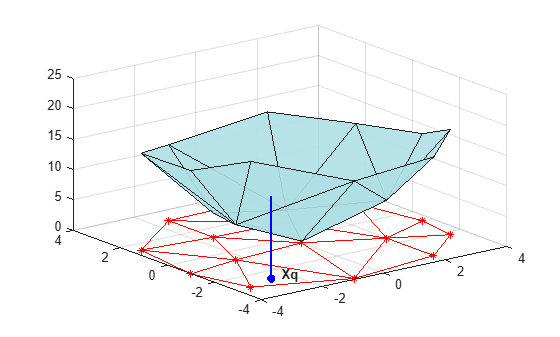This step generally involves traversing of the triangulation data structure to find the triangle that encloses the query point. Once you find the point, the subsequent steps to compute the value depend on the interpolation method. You could compute the nearest point in the neighborhood and use the value at that point (the nearest-neighbor interpolation method). You could also compute the weighted sum of values of the three vertices of the enclosing triangle (the linear interpolation method). These methods and their variants are covered in texts and references on scattered data interpolation.

Though the illustration highlights 2-D interpolation, you can apply this technique to higher dimensions. In more general terms, given a set of points `X` and corresponding values `V`, you can construct an interpolant of the form `V = F(X)`. You can evaluate the interpolant at a query point `Xq`, to give `Vq = F(Xq)`. This is a single-valued function; for any query point `Xq` within the convex hull of `X`, it will produce a unique value `Vq`. The sample data is assumed to respect this property in order to produce a satisfactory interpolation.

MATLAB® provides two ways to perform triangulation-based scattered data interpolation:

The `griddata` function supports 2-D scattered data interpolation. The `griddatan` function supports scattered data interpolation in N-D; however, it is not practical in dimensions higher than 6-D for moderate to large point sets, due to the exponential growth in memory required by the underlying triangulation.

The `scatteredInterpolant` class supports scattered data interpolation in 2-D and 3-D space. Use of this class is encouraged as it is more efficient and readily adapts to a wider range of interpolation problems.

### Interpolating Scattered Data Using griddata and griddatan

The `griddata` and `griddatan` functions take a set of sample points, `X`, corresponding values, `V`, and query points, `Xq`, and return the interpolated values, `Vq`. The calling syntax is similar for each function; the primary distinction is the 2-D / 3–D `griddata` function lets you define the points in terms of `X`, `Y` / `X`, `Y`, `Z` coordinates. These two functions interpolate scattered data at predefined grid-point locations; the intent is to produce gridded data, hence the name. Interpolation is more general in practice. You might want to query at arbitrary locations within the convex hull of the points.

This example shows how the `griddata` function interpolates scattered data at a set of grid points and uses this gridded data to create a contour plot.

Plot the `seamount` data set (a seamount is an underwater mountain). The data set consists of a set of longitude (`x`) and latitude (`y`) locations, and corresponding `seamount` elevations (`z`) measured at those coordinates.

```load seamount plot3(x,y,z,'.','markersize',12) xlabel('Longitude') ylabel('Latitude') zlabel('Depth in Feet') grid on```Use `meshgrid` to create a set of 2-D grid points in the longitude-latitude plane and then use `griddata` to interpolate the corresponding depth at those points.

```figure [xi,yi] = meshgrid(210.8:0.01:211.8, -48.5:0.01:-47.9); zi = griddata(x,y,z,xi,yi); surf(xi,yi,zi); xlabel('Longitude') ylabel('Latitude') zlabel('Depth in Feet')```Now that the data is in a gridded format, compute and plot the contours.

```figure [c,h] = contour(xi,yi,zi); clabel(c,h); xlabel('Longitude') ylabel('Latitude')```You can also use `griddata` to interpolate at arbitrary locations within the convex hull of the dataset. For example, the depth at coordinates (211.3, -48.2) is given by:

`zi = griddata(x,y,z, 211.3, -48.2);`

The underlying triangulation is computed each time the `griddata` function is called. This can impact performance if the same data set is interpolated repeatedly with different query points. The `scatteredInterpolant` class described in Interpolating Scattered Data Using the scatteredInterpolant Class is more efficient in this respect.

MATLAB software also provides `griddatan` to support interpolation in higher dimensions. The calling syntax is similar to `griddata`.

### scatteredInterpolant Class

The `griddata` function is useful when you need to interpolate to find the values at a set of predefined grid-point locations. In practice, interpolation problems are often more general, and the `scatteredInterpolant` class provides greater flexibility. The class has the following advantages:

• It produces an interpolating function that can be queried efficiently. That is, the underlying triangulation is created once and reused for subsequent queries.

• The interpolation method can be changed independently of the triangulation.

• The values at the data points can be changed independently of the triangulation.

• Data points can be incrementally added to the existing interpolant without triggering a complete recomputation. Data points can also be removed and moved efficiently, provided the number of points edited is small relative to the total number of sample points.

• It provides extrapolation functionality for approximating values at points that fall outside the convex hull. See Extrapolating Scattered Data for more information.

`scatteredInterpolant` provides the following interpolation methods:

• `'nearest'` — Nearest-neighbor interpolation, where the interpolating surface is discontinuous.

• `'linear'` — Linear interpolation (default), where the interpolating surface is C0 continuous.

• `'natural'` — Natural-neighbor interpolation, where the interpolating surface is C1 continuous except at the sample points.

The `scatteredInterpolant` class supports scattered data interpolation in 2-D and 3-D space. You can create the interpolant by calling `scatteredInterpolant` and passing the point locations and corresponding values, and optionally the interpolation and extrapolation methods. See the `scatteredInterpolant` reference page for more information about the syntaxes you can use to create and evaluate a `scatteredInterpolant`.

### Interpolating Scattered Data Using the scatteredInterpolant Class

This example shows how to use `scatteredInterpolant` to interpolate a scattered sampling of the `peaks` function.

Create the scattered data set.

```X = -3 + 6.*gallery('uniformdata',[250 2],0); V = peaks(X(:,1),X(:,2));```

Create the interpolant.

`F = scatteredInterpolant(X,V)`
```F = scatteredInterpolant with properties: Points: [250x2 double] Values: [250x1 double] Method: 'linear' ExtrapolationMethod: 'linear' ```

The `Points` property represents the coordinates of the data points, and the `Values` property represents the associated values. The `Method` property represents the interpolation method that performs the interpolation. The `ExtrapolationMethod` property represents the extrapolation method used when query points fall outside the convex hull.

You can access the properties of `F` in the same way you access the fields of a `struct`. For example, use `F.Points` to examine the coordinates of the data points.

Evaluate the interpolant.

`scatteredInterpolant` provides subscripted evaluation of the interpolant. It is evaluated the same way as a function. You can evaluate the interpolant as follows. In this case, the value at the query location is given by `Vq`. You can evaluate at a single query point:

`Vq = F([1.5 1.25])`
```Vq = 1.3966 ```

You can also pass individual coordinates:

`Vq = F(1.5, 1.25)`
```Vq = 1.3966 ```

You can evaluate at a vector of point locations:

```Xq = [0.5 0.25; 0.75 0.35; 1.25 0.85]; Vq = F(Xq)```
```Vq = 3×1 1.0880 1.8127 2.3472 ```

You can evaluate `F` at grid point locations and plot the result.

```[Xq,Yq] = meshgrid(-2.5:0.125:2.5); Vq = F(Xq,Yq); surf(Xq,Yq,Vq); xlabel('X','fontweight','b'), ylabel('Y','fontweight','b'); zlabel('Value - V','fontweight','b'); title('Linear Interpolation Method','fontweight','b');```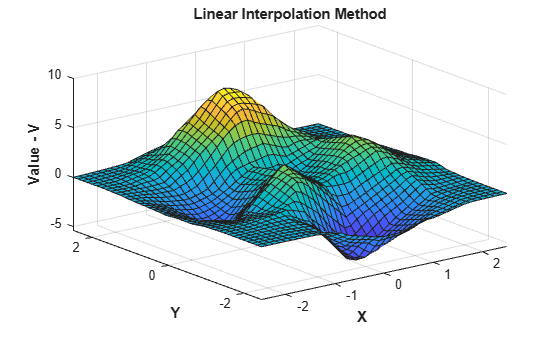Change the interpolation method.

You can change the interpolation method on the fly. Set the method to `'nearest'`.

`F.Method = 'nearest';`

Reevaluate and plot the interpolant as before.

```Vq = F(Xq,Yq); figure surf(Xq,Yq,Vq); xlabel('X','fontweight','b'),ylabel('Y','fontweight','b') zlabel('Value - V','fontweight','b') title('Nearest neighbor Interpolation Method','fontweight','b');```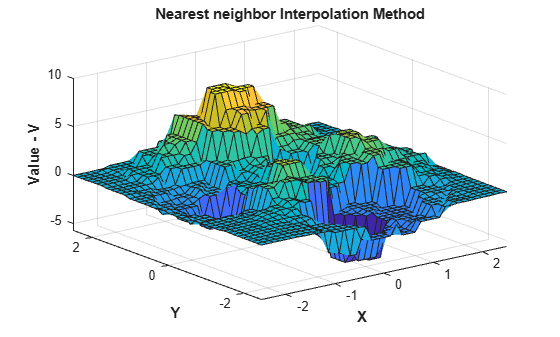Change the interpolation method to natural neighbor, reevaluate, and plot the results.

```F.Method = 'natural'; Vq = F(Xq,Yq); figure surf(Xq,Yq,Vq); xlabel('X','fontweight','b'),ylabel('Y','fontweight','b') zlabel('Value - V','fontweight','b') title('Natural neighbor Interpolation Method','fontweight','b');```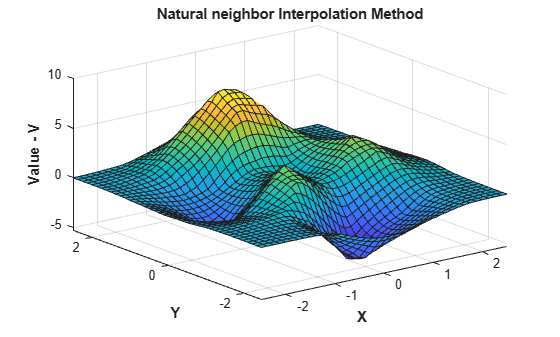Replace the values at the sample data locations.

You can change the values `V` at the sample data locations, `X`, on the fly. This is useful in practice as some interpolation problems may have multiple sets of values at the same locations. For example, suppose you want to interpolate a 3-D velocity field that is defined by locations (`x`, `y`, `z`) and corresponding componentized velocity vectors (`Vx`, `Vy`, `Vz`). You can interpolate each of the velocity components by assigning them to the values property (`V`) in turn. This has important performance benefits, because it allows you to reuse the same interpolant without incurring the overhead of computing a new one each time.

The following steps show how to change the values in our example. You will compute the values using the expression, $v=x{e}^{-{x}^{2}-{y}^{2}}$.

```V = X(:,1).*exp(-X(:,1).^2-X(:,2).^2); F.Values = V;```

Evaluate the interpolant and plot the result.

```Vq = F(Xq,Yq); figure surf(Xq,Yq,Vq); xlabel('X','fontweight','b'), ylabel('Y','fontweight','b') zlabel('Value - V','fontweight','b') title('Natural neighbor interpolation of v = x.*exp(-x.^2-y.^2)')```This performs an efficient update as opposed to a complete recomputation using the augmented data set.

When adding sample data, it is important to add both the point locations and the corresponding values.

Continuing the example, create new sample points as follows:

```X = -1.5 + 3.*rand(100,2); V = X(:,1).*exp(-X(:,1).^2-X(:,2).^2);```

Add the new points and corresponding values to the triangulation.

```F.Points(end+(1:100),:) = X; F.Values(end+(1:100)) = V;```

Evaluate the refined interpolant and plot the result.

```Vq = F(Xq,Yq); figure surf(Xq,Yq,Vq); xlabel('X','fontweight','b'), ylabel('Y','fontweight','b'); zlabel('Value - V','fontweight','b');```Remove data from the interpolant.

You can incrementally remove sample data points from the interpolant. You also can remove data points and corresponding values from the interpolant. This is useful for removing spurious outliers.

When removing sample data, it is important to remove both the point location and the corresponding value.

Remove the 25th point.

```F.Points(25,:) = []; F.Values(25) = [];```

Remove points 5 to 15.

```F.Points(5:15,:) = []; F.Values(5:15) = [];```

Retain 150 points and remove the rest.

```F.Points(150:end,:) = []; F.Values(150:end) = [];```

This creates a coarser surface when you evaluate and plot:

```Vq = F(Xq,Yq); figure surf(Xq,Yq,Vq); xlabel('X','fontweight','b'), ylabel('Y','fontweight','b'); zlabel('Value - V','fontweight','b'); title('Interpolation of v = x.*exp(-x.^2-y.^2) with sample points removed')```### Interpolation of Complex Scattered Data

This example shows how to interpolate scattered data when the value at each sample location is complex.

Create the sample data.

```X = -3 + 6 .* gallery('uniformdata',[250 2],0); V = complex(X(:,1).*X(:,2), X(:,1).^2 + X(:,2).^2);```

Create the interpolant.

`F = scatteredInterpolant(X,V);`

Create a grid of query points and evaluate the interpolant at the grid points.

```[Xq,Yq] = meshgrid(-2.5:0.125:2.5); Vq = F(Xq,Yq);```

Plot the real component of `Vq`.

```VqReal = real(Vq); figure surf(Xq,Yq,VqReal); xlabel('X'); ylabel('Y'); zlabel('Real Value - V'); title('Real Component of Interpolated Value');```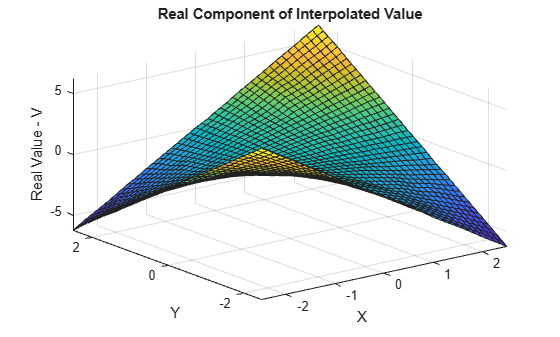Plot the imaginary component of `Vq`.

```VqImag = imag(Vq); figure surf(Xq,Yq,VqImag); xlabel('X'); ylabel('Y'); zlabel('Imaginary Value - V'); title('Imaginary Component of Interpolated Value');```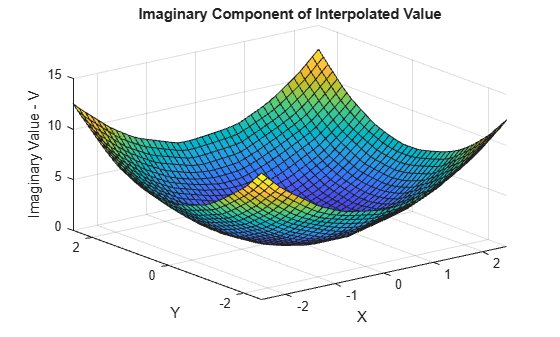### Addressing Problems in Scattered Data Interpolation

Many of the illustrative examples in the previous sections dealt with the interpolation of point sets that were sampled on smooth surfaces. In addition, the points were relatively uniformly spaced. For example, clusters of points were not separated by relatively large distances. In addition, the interpolant was evaluated well within the convex hull of the point locations.

When dealing with real-world interpolation problems the data may be more challenging. It may come from measuring equipment that is likely to produce inaccurate readings or outliers. The underlying data may not vary smoothly, the values may jump abruptly from point to point. This section provides you with some guidelines to identify and address problems with scattered data interpolation.

#### Input Data Containing NaNs

You should preprocess sample data that contains `NaN` values to remove the `NaN` values as this data cannot contribute to the interpolation. If a `NaN` is removed, the corresponding data values/coordinates should also be removed to ensure consistency. If `NaN` values are present in the sample data, the constructor will error when called.

The following example illustrates how to remove `NaN`s.

Create some data and replace some entries with `NaN`:

```x = rand(25,1)*4-2; y = rand(25,1)*4-2; V = x.^2 + y.^2; x(5) = NaN; x(10) = NaN; y(12) = NaN; V(14) = NaN;```
This code errors:
`F = scatteredInterpolant(x,y,V);`
Instead, find the sample point indices of the `NaN`s and then construct the interpolant:
```nan_flags = isnan(x) | isnan(y) | isnan(V); x(nan_flags) = []; y(nan_flags) = []; V(nan_flags) = []; F = scatteredInterpolant(x,y,V);```
The following example is similar if the point locations are in matrix form. First, create data and replace some entries with `NaN` values.
```X = rand(25,2)*4-2; V = X(:,1).^2 + X(:,2).^2; X(5,1) = NaN; X(10,1) = NaN; X(12,2) = NaN; V(14) = NaN;```
This code errors:
`F = scatteredInterpolant(X,V);`
Find the sample point indices of the NaN and then construct the interpolant:
```nan_flags = isnan(X(:,1)) | isnan(X(:,2)) | isnan(V); X(nan_flags,:) = []; V(nan_flags) = []; F = scatteredInterpolant(X,V);```

#### Interpolant Outputs NaN Values

`griddata` and `griddatan` return `NaN` values when you query points outside the convex hull using the `'linear'` or `'natural'` methods. However, you can expect numeric results if you query the same points using the `'nearest'` method. This is because the nearest neighbor to a query point exists both inside and outside the convex hull.

If you want to compute approximate values outside the convex hull, you should use `scatteredInterpolant`. See Extrapolating Scattered Data for more information.

#### Handling Duplicate Point Locations

Input data is rarely “perfect” and your application could have to handle duplicate data point locations. Two or more data points at the same location in your data set can have different corresponding values. In this scenario, `scatteredInterpolant` merges the points and computes the average of the corresponding values. This example shows how `scatteredInterpolant` performs an interpolation on a data set with duplicate points.

1. Create some sample data that lies on a planar surface:

```x = rand(100,1)*6-3; y = rand(100,1)*6-3; V = x + y; ```

2. Introduce a duplicate point location by assigning the coordinates of point 50 to point 100:

```x(50) = x(100); y(50) = y(100);```

3. Create the interpolant. Notice that `F` contains 99 unique data points:

`F = scatteredInterpolant(x,y,V)`

4. Check the value associated with the 50th point:

`F.Values(50)`

This value is the average of the original 50th and 100th value, as these two data points have the same location:

`(V(50)+V(100))/2`
In this scenario the interpolant resolves the ambiguity in a reasonable manner. However in some instances, data points can be close rather than coincident, and the values at those locations can be different.

In some interpolation problems, multiple sets of sample values might correspond to the same locations. For example, a set of values might be recorded at the same locations at different periods in time. For efficiency, you can interpolate one set of readings and then replace the values to interpolate the next set.

Always use consistent data management when replacing values in the presence of duplicate point locations. Suppose you have two sets of values associated with the 100 data point locations and you would like to interpolate each set in turn by replacing the values.

1. Consider two sets of values:

```V1 = x + 4*y; V2 = 3*x + 5*y ```

2. Create the interpolant. `scatteredInterpolant` merges the duplicate locations and the interpolant contains 99 unique sample points:

`F = scatteredInterpolant(x,y,V1)`
Replacing the values directly via `F.Values = V2` means assigning 100 values to 99 samples. The context of the previous merge operation is lost; the number of sample locations will not match the number of sample values. The interpolant will require the inconsistency to be resolved to support queries.

In this more complex scenario, it is necessary to remove the duplicates prior to creating and editing the interpolant. Use the `unique` function to find the indices of the unique points. `unique` can also output arguments that identify the indices of the duplicate points.

```[~, I, ~] = unique([x y],'first','rows'); I = sort(I); x = x(I); y = y(I); V1 = V1(I); V2 = V2(I); F = scatteredInterpolant(x,y,V1)```
Now you can use `F` to interpolate the first data set. Then you can replace the values to interpolate the second data set.
`F.Values = V2;`

#### Achieving Efficiency When Editing a scatteredInterpolant

`scatteredInterpolant` allows you to edit the properties representing the sample values (`F.Values`) and the interpolation method (`F.Method`). Since these properties are independent of the underlying triangulation, the edits can be performed efficiently. However, like working with a large array, you should take care not to accidentally create unnecessary copies when editing the data. Copies are made when more than one variable references an array and that array is then edited.

A copy is not made in the following:

```A1 = magic(4) A1(4,4) = 11 ```
However, a copy is made in this scenario because the array is referenced by another variable. The arrays `A1` and `A2` can no longer share the same data once the edit is made:
```A1 = magic(4) A2 = A1 A1(4,4) = 32```
Similarly, if you pass the array to a function and edit the array within that function, a deep copy may be made depending on how the data is managed. `scatteredInterpolant` contains data and it behaves like an array—in MATLAB language, it is called a value object. The MATLAB language is designed to give optimum performance when your application is structured into functions that reside in files. Prototyping at the command line may not yield the same level of performance.

The following example demonstrates this behavior, but it should be noted that performance gains in this example do not generalize to other functions in MATLAB.

This code does not produce optimal performance:

```x = rand(1000000,1)*4-2; y = rand(1000000,1)*4-2; z = x.*exp(-x.^2-y.^2); tic; F = scatteredInterpolant(x,y,z); toc tic; F.Values = 2*z; toc```
You can place the code in a function file to execute it more efficiently.

When MATLAB executes a program that is composed of functions that reside in files, it has a complete picture of the execution of the code; this allows MATLAB to optimize for performance. When you type the code at the command line, MATLAB cannot anticipate what you are going to type next, so it cannot perform the same level of optimization. Developing applications through the creation of reusable functions is general and recommended practice, and MATLAB will optimize the performance in this setting.

#### Interpolation Results Poor Near the Convex Hull

The Delaunay triangulation is well suited to scattered data interpolation problems because it has favorable geometric properties that produce good results. These properties are:

• The rejection of sliver-shaped triangles/tetrahedra in favor of more equilateral-shaped ones.

• The empty circumcircle property that implicitly defines a nearest-neighbor relation between the points.

The empty circumcircle property ensures the interpolated values are influenced by sample points in the neighborhood of the query location. Despite these qualities, in some situations the distribution of the data points may lead to poor results and this typically happens near the convex hull of the sample data set. When the interpolation produces unexpected results, a plot of the sample data and underlying triangulation can often provide insight into the problem.

This example shows an interpolated surface that deteriorates near the boundary.

Create a sample data set that will exhibit problems near the boundary.

```t = 0.4*pi:0.02:0.6*pi; x1 = cos(t)'; y1 = sin(t)'-1.02; x2 = x1; y2 = y1*(-1); x3 = linspace(-0.3,0.3,16)'; y3 = zeros(16,1); x = [x1;x2;x3]; y = [y1;y2;y3];```

Now lift these sample points onto the surface $z={x}^{2}+{y}^{2}$ and interpolate the surface.

```z = x.^2 + y.^2; F = scatteredInterpolant(x,y,z); [xi,yi] = meshgrid(-0.3:.02:0.3, -0.0688:0.01:0.0688); zi = F(xi,yi); mesh(xi,yi,zi) xlabel('X','fontweight','b'), ylabel('Y','fontweight','b') zlabel('Value - V','fontweight','b') title('Interpolated Surface');```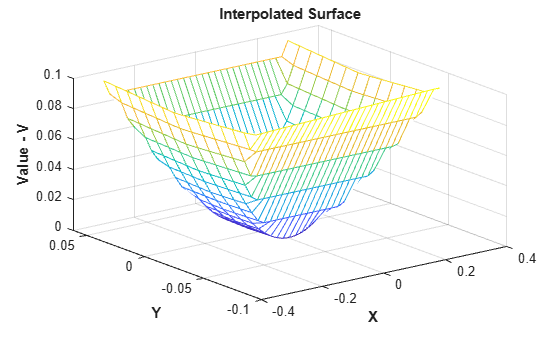The actual surface is:

```zi = xi.^2 + yi.^2; figure mesh(xi,yi,zi) title('Actual Surface')```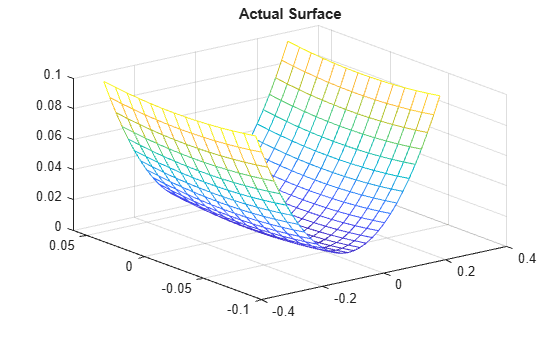To understand why the interpolating surface deteriorates near the boundary, it is helpful to look at the underlying triangulation:

```dt = delaunayTriangulation(x,y); figure plot(x,y,'*r') axis equal hold on triplot(dt) plot(x1,y1,'-r') plot(x2,y2,'-r') title('Triangulation Used to Create the Interpolant') hold off```The triangles within the red boundaries are relatively well shaped; they are constructed from points that are in close proximity and the interpolation works well in this region. Outside the red boundary, the triangles are sliver-like and connect points that are remote from each other. There is not sufficient sampling to accurately capture the surface, so it is not surprising that the results in these regions are poor. In 3-D, visual inspection of the triangulation gets a bit trickier, but looking at the point distribution can often help illustrate potential problems.

The MATLAB® 4 `griddata` method, `'v4'`, is not triangulation-based and is not affected by deterioration of the interpolation surface near the boundary.

```[xi,yi] = meshgrid(-0.3:.02:0.3, -0.0688:0.01:0.0688); zi = griddata(x,y,z,xi,yi,'v4'); mesh(xi,yi,zi) xlabel('X','fontweight','b'), ylabel('Y','fontweight','b') zlabel('Value - V','fontweight','b') title('Interpolated surface from griddata with v4 method','fontweight','b');```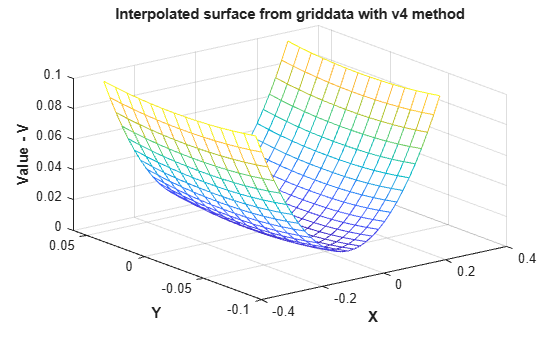The interpolated surface from `griddata` using the `'v4'` method corresponds to the expected actual surface.